[中文] Search Internet Search HelloDBA
Oracle Technology Site. email: fuyuncat@gmail.com  MSN: fuyuncat@hotmail.com# CBO Statistics data gathering and internal arithmetic analysis -- when will gather histogram data

[中文]

Author:  fuyuncat

Source:  www.HelloDBA.com

Date:  2010-01-07 09:47:08

Share to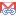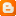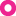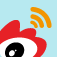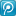Statistics data and histogram data are the basic data for Cost Base Optimizer to calculate the cost. They are correct or not, decide if optimizer can get the best execution plan. I will analyze the statistics data gathering internal process and arithmetic in following papers.

## 1. Will column's Histogram data be gathtered?

During table statistics data gathering, there are several factors will affect whether oracle will gather and store column's histogram data.

The first one, of course, is that have the columns be specified in analyaze statement or METHOD_OPT of dbms_stats procedure.

•   FOR ALL COLUMNS;
•   FOR ALL INDEXED COLUMNS;
•   FOR ALL HIDDEN COLUMNS;
•   FOR COLUMNS <COL1> <COL2> ... ;

For those specified columns, oracle need get below 2 properties for following judgement.

•  Unique Column: The column is the ONLY ONE column in unique key;
•  Indexed Column: The column is the LEADING column in at least one index.

SQL代码
1. -- Here check the column in table/cluster is unique or indexed
2. HELLODBA.COM>SELECT COUNT(UNQ) UNQ, COUNT(PFX) PFX FROM
3.   2   (SELECT /*+ first_rows(1) leading(cc) */
4.   3      CD.TYPE# UNQ, NULL PFX
5.   4    FROM SYS.CCOL\$ CC, SYS.CDEF\$ CD
6.   5    WHERE CC.OBJ# = 20
7.   6      AND CC.INTCOL# = 1
8.   7      AND CD.CON# = CC.CON#
9.   8      AND CD.OBJ# = CC.OBJ#
10.   9      AND CD.ENABLED IS NOT NULL
11.  10      AND CD.INTCOLS = 1
12.  11      AND CD.TYPE# IN (2,3)
13.  12      AND BITAND(CD.DEFER, 2+4) = 4
14.  13      AND ROWNUM < 2
15.  14    UNION ALL
16.  15    SELECT /*+ first_rows(1) leading(i) */
17.  16      CASE WHEN I.INTCOLS = 1 AND BITAND(I.PROPERTY,1) = 1 THEN 3
18.  17        ELSE NULL END UNQ,
19.  18      CASE WHEN IC.POS# = 1 THEN 1 ELSE NULL END PFX
20.  19    FROM SYS.IND\$ I, SYS.ICOL\$ IC
21.  20    WHERE I.BO# = 20
22.  21      AND IC.INTCOL# = 1
23.  22      AND I.BO# = IC.BO#
24.  23      AND I.OBJ# = IC.OBJ#
25.  24      AND BITAND(I.FLAGS,1025) = 0
26.  25      AND ROWNUM < 2
27.  26   );
28.
29.        UNQ        PFX
30. ---------- ----------
31.          0          1

Here following the important factor it the bucket size setting. Oracle will set 2 kinds of bit flag to decide whether gather histogram data, according to requirement in different setting.

•   -AUTO: Oracle determines the columns to collect histograms based on data distribution and the workload of the columns
•     The columns be used for equality prediction or equality join prediction in recent 3 minutes, will set EUAL_PRED bit;
•     The columns be used for range prediction or like prediction in recent 3 minutes, will set RANGE_PRED bit;
•     The maximum bucket number is the distinct value number from sample data, and should not larger than 254.
•   -SKEWONLY: Oracle determines the columns to collect histograms based on the data distribution of the columns
•     The Non-unqiue columns will set EUAL_PRED bit;
•     All of the columns will set RANGE_PRED bit;
•     The maximum bucket number is the distinct value number from sample data, and should not larger than 254.
•   -REPEAT: Collects histograms only on the columns that already have histograms.
•     All of the columns that already have histograms will set EUAL_PRED and RANGE_PRED bits;
•     The maximum bucket number is original bucket number;
•   -<Number>: User specified size
•     All of the columns will set EUAL_PRED and RANGE_PRED bits;
•     The maximum bucket number is the specified size;
•   -Not set: means use default setting
•     All of the columns will set EUAL_PRED and RANGE_PRED bits;
•     The maximum bucket number is the default size, 75;

Only when EUAL_PRED and RANGE_PRED bits are set, the column will set GATHER_HISTOGRAM bit.

Note: we can get the column recent usage timestamp and prediction times by querying COL_USAGE\$.

SQL代码
1. HELLODBA.COM>select timestamp last_usage_time, equality_preds, equijoin_preds, range_preds, like_preds from COL_USAGE\$ w;
2.
3. LAST_USAG EQUALITY_PREDS EQUIJOIN_PREDS RANGE_PREDS LIKE_PREDS
4. --------- -------------- -------------- ----------- ----------
5. 07-JAN-10          42745           1713           0          0

For those columns has set GATHER_HISTOGRAM bit, oracle will create their histogram data. However, that not means the histogram data will be stored correpondly, which should be decided by following factors.

There are 2 methods to create histogram data, Frequency and Height Balanced. We will discuss how does oracle choose which one in next paper.

There are the differences of these 2 kinds of histogram data.

• Frequency Histogram: Each value of the column will repet frequently. Oracle create a bucket for each value. Data will be groupped by values. It's normally for those columns with low number of distinct values;
• Height Balanced Histogram: Height of each bucket is close, means balanced. Data will be groupped by bucket number. It's normally for those columns wiht high number of distinct values. However, there may be few values with high frequency in such column, and they may take multiple buckets. Those buckets will be merged (compressed), and such values are named popular values.

For the frequency histogram, oracle will store the histogram data under below conditions:

• The column has set EUAL_PRED bit, and it's actual bucket number less than sample size;
• The column has set RANGE_PRED bit, and oracle will check the data distribution by internal arithmetic. I will demo the arithmetic by a perl script later.

For the Height Balanced histogram, oracle will store the histogram data under below conditions:

• The column has set EUAL_PRED bit, there is popular values in this column;
• The column has set RANGE_PRED bit, and oracle will check the data distribution by internal arithmetic, which is the same arithmetic in frequency histogram.

SQL代码
1. HELLODBA.COM>host perl Range_Skew_test.pl
2. dif: 16
3. th: 16
4.   The 0 (0) bucket:
5.   rc: 0
6.   The 1 (30) bucket:
7.    In step 16 ... 32
8.    Totc: 4
9.   rc: 0
10.   The 2 (33) bucket:
11.    In step 32 ... 48
12.    Totc: 68
13.   rc: 0
14.   The 3 (38) bucket:
15.   rc: 13
16. Final totc: 68;
17. Check value(totc/arrcnt): 13.6
18. Checking passed, need be kept.